Clutch Prep is now a part of Pearson

# The Ideal Gas Law

See all sections
Sections
Pressure Units
The Ideal Gas Law
The Ideal Gas Law Derivations
The Ideal Gas Law Applications
Chemistry Gas Laws
Chemistry Gas Laws: Combined Gas Law
Mole Fraction
Partial Pressure
The Ideal Gas Law: Molar Mass
The Ideal Gas Law: Density
Gas Stoichiometry
Standard Temperature and Pressure
Effusion
Root Mean Square Speed
Kinetic Energy of Gases
Maxwell-Boltzmann Distribution
Velocity Distributions
Kinetic Molecular Theory
Van der Waals Equation
Boyle's Law (IGNORE)
Charles Law (IGNORE)
Ideal Gas Law (IGNORE)Jules Bruno

The Simple Gas Laws represent theories and equations that try to relate together pressure, temperature, volume and the amount of a gas when discussing their chemical properties and behaviors.

Boyle’s Law

Boyle’s Law, also known as Mariotte’s Law, the Boyle-Mariotte Law or Pressure-Volume Law, states that pressure (P) and volume (V) are inversely proportionally, which basically means they are opposites of one another, at constant moles (n) and temperature (T).

This pressure-volume relationship is commonly displayed in the following example of a closed container. Pressure represents the downward force applied to the container while volume represents the free space within it.Boyle's Law (Volume & Pressure)

When plotting this inverse pressure-volume relationship onto a chart you obtain the following: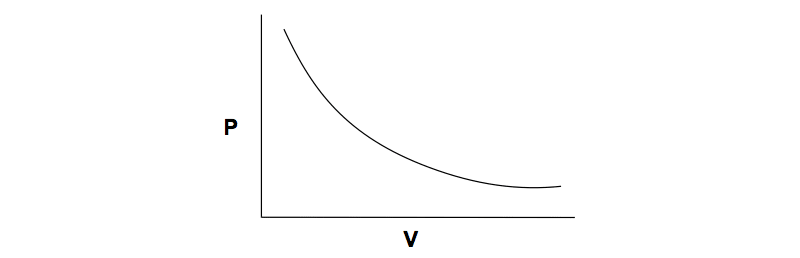Pressure-Volume Plot

The inverse relationship between pressure and volume at a constant mass for a gas at a fixed temperature is illustrated by the expression:Volume & Pressure (Inverse Relationship)

Boyle’s Law Formula

The Ideal Gas Law is stated as: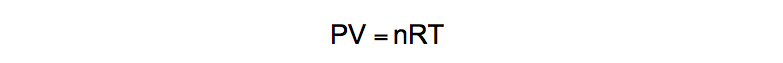Ideal Gas Law

If moles (n) and temperature (T) are held constant then the formula simplifies into:Boyle's Law (PV = Constant)

When dealing with two sets of data (2 pressures and 2 volumes) the Boyle’s Law formula becomes:Boyle's Law Formula (P1V1 = P2V2)

Now let’s use it in a practice problem.

PRACTICE: If an unknown gas were confined within a 5.15 L container at 3.20 atm, what would be the pressure if the volume were expanded to 11.23 L?

STEP 1: Identify the variables given.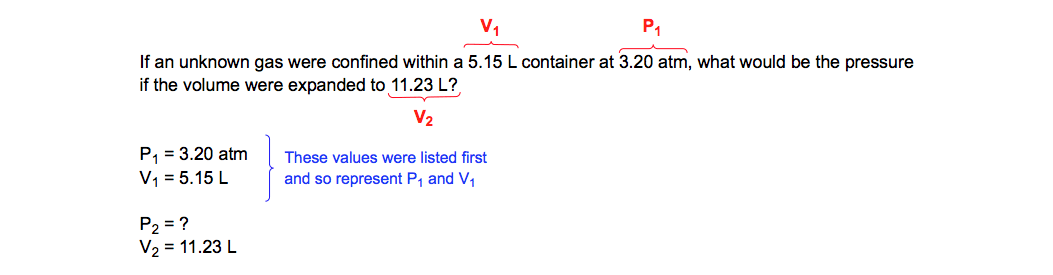Boyle's Law (Identifying Variables)

STEP 2: Isolate the missing variable for the second pressure (P2).Boyle's Law (Solving for P2)

Charles’ Law

Charles Law, also known as the Temperature-Volume Law, states that volume (V) and temperature (T) are directly proportionally as long as pressure (P) and moles (n) are held constant. This temperature-volume relationship is commonly displayed by the application and removal of heat from a closed container with a moveable piston.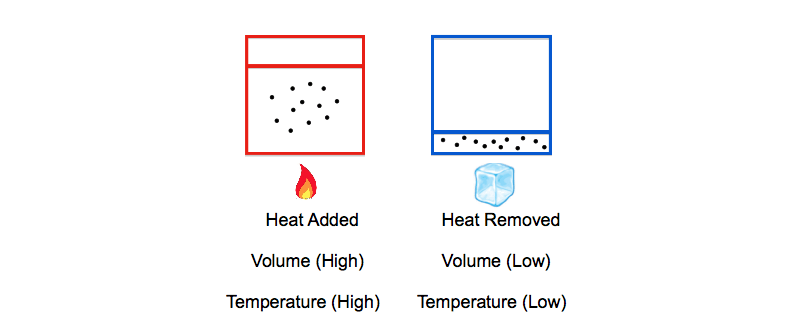Charles' Law (Volume & Temperature)

Being directly proportional means that as the temperature increases then the volume would also increase. This direct temperature-volume relationship can be plotted onto a chart and provide the following: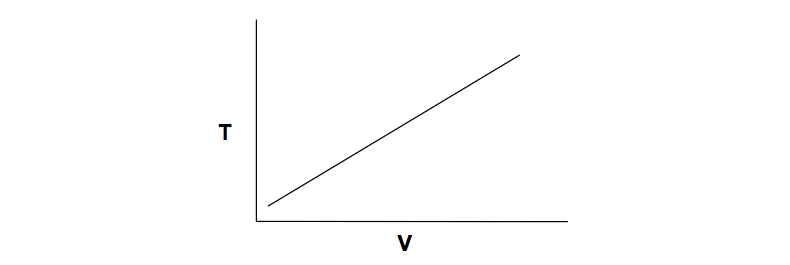Temperature-Volume Plot

The direct relationship between temperature and volume at constant moles and pressure is illustrated by the expression:Volume & Temperature (Direct Relationship)

Charles’ Law Formula

By rearranging the Ideal Gas Law we can isolate V and T:Derived Formula (Charles' Law)

If moles (n) and pressure (P) are held constant then the formula simplifies into:Charles' Law (V/T = Constant)

Incorporating the two sets of data (2 volumes, 2 temperatures) produces the Charles’ Law formula as:Charles' Law Formula (V1 / T1 = V2 / T2)

PRACTICE: If a 2.2 L container filled with gas at a temperature of 18.0 °C is heated to a temperature of 38.0 °C, what is the new volume of the container (in liters)?

STEP 1: Identify the variables given.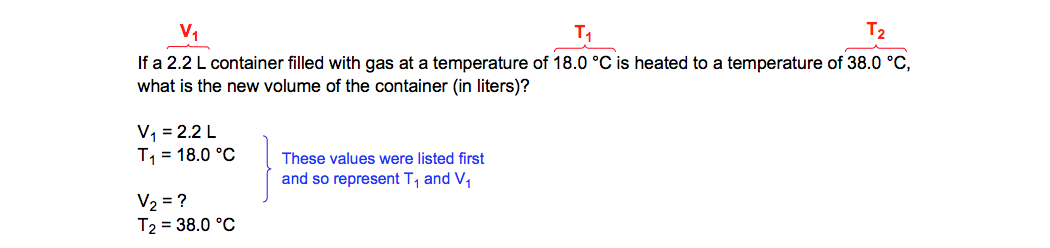Charles' Law (Identifying Variables)

STEP 2: Gas behavior is examined under absolute temperature conditions and so we must convert all temperatures from Celsius to Kelvin.Temperature Conversions (T1 & T2)

STEP 3: Plug the given values into Charles’ Law formula.Charles' Law (Plugging in values)

STEP 4: Perform cross-multiplication between the two ratios.Charles' Law (Cross Multiplication)

STEP 5: Isolate the missing variable for the second volume (V2).Charles' Law (Solving for V2)

Avogadro’s Law, also known as the Mole-Volume Law or Volume Amount Law, states that volume (V) and moles (n) are directly proportional as long as pressure (P) and temperature (T) are held constant. This mole-volume relationship is depicted with the addition or removal of gas molecules from a closed container with a moveable piston.Avogadro's Law (Moles & Volume)

As more and more gas molecules are pumped into the container they push up against the moveable piston and thereby increase the volume inside the container. This direct mole-volume relationship can be plotted onto a chart and provide the following:Moles-Volume Plot

The direct relationship between the number of moles and volume at constant temperature and pressure is illustrated by the expression:Volume & moles (Direct Relationship)

By rearranging the Ideal Gas Law we can isolate V and n:Derived Formula (Avogadro's Law)

If temperature (T) and pressure (P) are held constant then the formula simplifies into:Avogadro's Law (V/n = Constant)

Incorporating the two sets of data (2 volumes, 2 moles) produces the Avogadro’s Law formula as:Avogadro's Law (V1/n1 = V2/n2)

PRACTICE: How many moles will a sample of F2 gas occupy in a 2.50 L container, if 3.21 moles of F2 have a volume of 53.2 L?

STEP 1: Identify the variables given.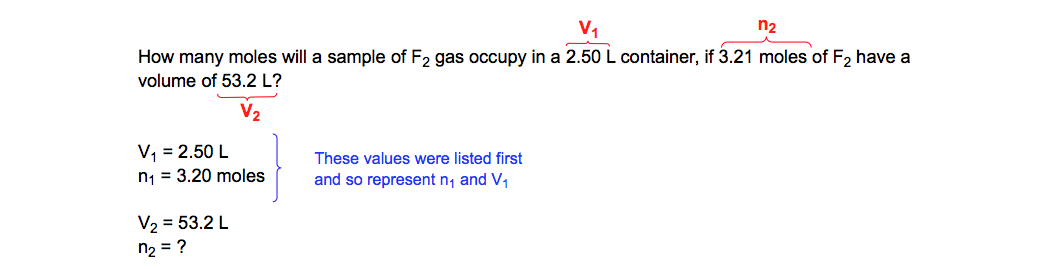Avogadro's Law (Identifying Variables)

STEP 2: Plug the given values into Avogadro’s Law formula.Avogadro's Law (Plugging in values)

STEP 3: Perform cross-multiplication between the two ratios.Avogadro's Law (Cross Multiplication)

STEP 4: Isolate the missing variable for the second moles (n2).Avogadro's Law (Solving for n2)

Gay-Lussac’s Law

This sometimes overlooked simple gas law, because it doesn’t involve a changing volume like the previous 3, states that pressure (P) and temperature (T) are directly proportional as long as volume (V) and moles (n) are held constant. This pressure-temperature relationship is depicted with the addition or removal of heat from a rigid, non-flexible container.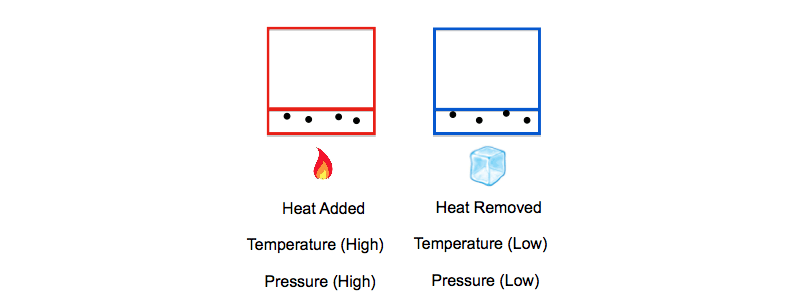Gay-Lussac's Law (Pressure & Temperature)

By increasing the temperature of a container the gas molecules will absorb the excess thermal energy and convert it into kinetic energy. With higher kinetic energies, the gas molecules will move faster and hit the walls of the container more frequently and with harder force. This in term leads to a higher overall pressure within the container.

This direct pressure-temperature relationship can be plotted onto a chart and provide the following:Pressure-Temperature Plot

The direct relationship between the pressure and temperature at constant volume and moles of gas is illustrated by the expression:Pressure & Temperature (Direct Relationship)

Gay-Lussac’s Law Formula

By rearranging the Ideal Gas Law we can isolate P and T:Derived Formula (Gay-Lussac's Law)

If volume (V) and moles of gas (n) are held constant then the formula simplifies into:Gay-Lussac's Law (P/T = Constant)

Incorporating the two sets of data (2 pressures, 2 temperatures) produces the Gay-Lussac’s Law formula as:Gay-Lussac's Law Formula (P1/T1 = P2/T2)

PRACTICE: If an unknown gas possesses a pressure of 800 mmHg when the temperature is 20 °C, what would be its pressure (in mmHg) when the temperature is increased to 110 °C

STEP 1: Identify the variables given.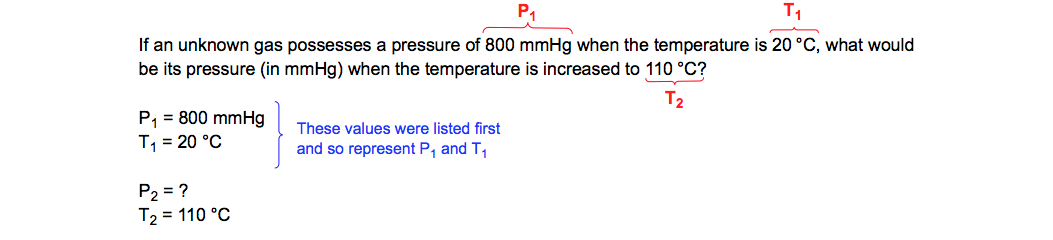Gay-Lussac's Law (Identifying Variables)

STEP 2: Convert the Celsius values into Kelvin.Avogadro's Law (Temperature Conversions)

STEP 3: Plug the given variables into the Gay-Lussac’s Law Formula.Gay-Lussac's Law Formula (Plugging in values)

STEP 4: Perform cross-multiplication between the two ratios.Gay-Lussac's Law (Cross Multiplication)

STEP 5: Isolate the missing variable for the second pressure (P2).Avogadro's Law (Solving for P2)

By combining the Simple Gas Laws we are able to create the Ideal Gas Law and the Combined Gas Law. Through these equations we can examine gas behaviors as well as their properties dealing with molar masspartial pressure, density, effusionspeed and velocityJules Bruno

Jules felt a void in his life after his English degree from Duke, so he started tutoring in 2007 and got a B.S. in Chemistry from FIU. He’s exceptionally skilled at making concepts dead simple and helping students in covalent bonds of knowledge.